Courses

# Test: Thin Cylinder Level - 3

## 10 Questions MCQ Test Engineering Mechanics | Test: Thin Cylinder Level - 3

Description
This mock test of Test: Thin Cylinder Level - 3 for Mechanical Engineering helps you for every Mechanical Engineering entrance exam. This contains 10 Multiple Choice Questions for Mechanical Engineering Test: Thin Cylinder Level - 3 (mcq) to study with solutions a complete question bank. The solved questions answers in this Test: Thin Cylinder Level - 3 quiz give you a good mix of easy questions and tough questions. Mechanical Engineering students definitely take this Test: Thin Cylinder Level - 3 exercise for a better result in the exam. You can find other Test: Thin Cylinder Level - 3 extra questions, long questions & short questions for Mechanical Engineering on EduRev as well by searching above.
QUESTION: 1

### A thin cylindrical shell made of steel, of diameter 250 mm, wall thickness 6 mm, length 1 m is subjected to internal pressure p such that maximum stress developed in the shell is 120 MPa. If E = 200 GPa and Poisson’s ratio is 0.3. The change in volume of the shell is ___________ cm3

Solution:

Maximum stress,

σc =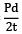= = 120 N/mm2

P=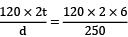= 5.76 N/mm2

E = 200000 N/mm2 , ν = 0.3

Axial strain,

εa =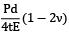=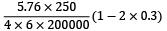= 1.2 × 10−4

Circumferential strain,

εc =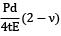=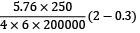= 5.1 × 10−4

Volumetric strain, εv = 2εc + εa

= 2 × 5.1 × 10−4 + 1.2 × 10−4

= 11.4 × 10−4

Original volume,

V =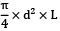=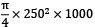= 49.0874 × 106 mm3

Change in volume, δV = εv × V

= 11.4 × 10−4 × 49.0874 × 106

= 55960 mm3 = 55.96 cm3

= 55.96 cc

Question_Type: 5

QUESTION: 2

### Match List-I with List-II and select the correct answer using the code given below the lists.List-I List –II(state of stress) (kind of loading)(a)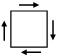1. Combined bending and torsion of circular shaft(b)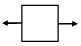2. Torsion of circular shaft(c)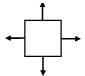3. Thin cylinder subjected to internal pressure(d)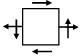4. Tie bar subjected to tensile forceCodes: a b c d

Solution: (i) Tie bar subjected to tensile force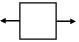(ii) Thin cylinder subjected to internal pressure σx = σ1 =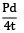σy = σ2 =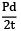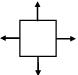(iii) Torsion of circular shaft is a case of pure shear.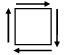(iv) Combined bending and torsion of circular shaft In this case both normal as well as shear stress act on the element.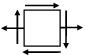QUESTION: 3

### A thin cylinder of radius r and thickness t when subjected to an internal hydrostatic pressure P causes a radial displacement u, then the tangential strain caused is

Solution: The thin walled pressure vessel expands when it is internally pressurized. This results in three principal strains, the circumferential strain (εc) or tangential strain in two perpendicular plane directions, and the radial strain εr .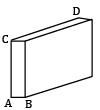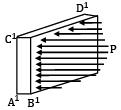Referring to the figure which shows strain of an element at the surface of the cylinder, we can write.

εc =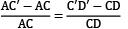and εr =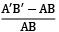To determine the amount by which the vessel expands, consider a circumference at radius r and which moves out with a displacementδr. From the definition of normal strain,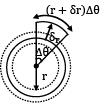εc =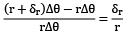here δr = u

⇒ εc =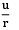This is the circumferential strain.

QUESTION: 4

A bronze cylinder of diameter 200 mm, wall thickness 8 mm is wound with steel wire of diameter 4 mm under initial tension of 20 N/mm2. The wire wound cylinder is subjected to internal pressure p such that resultant hoop and axial stresses developed in the cylinder are the same. What is the magnitude of p? Given Ew = 210 GPa, EC = 105 GPa, νc = 0.35.

Solution: σw = 20 N/mm2 dw = 4mm t = 8 mm

σc = Initial compressive stress in cylinder

=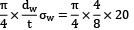= 7.854 N/mm2 (comp. )

Say internal pressure = P

Axial stress, σa′ =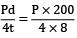= 6.25 P

Hoop stress = σc

σCR = (σc′ − 7.854) = 6.25 P, (as given) ⋯ ①

or σc′ = 6.25 P + 7.854 ⋯ ②

Using equilibrium and compatibility conditions

Pd = 2tσc′ +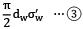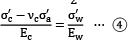From equation ④

σc′ − νcσ′c = σ′w ×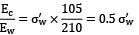or 6.25 P + 7.854 − 0.35 (6.25 P) = 0.5 σw

or 4.0625 P + 7.854 = 0.5 σw

σw′ = 8.125 P + 15.708

Putting the values σw′ and σc′ in equation ③

P × 200 = 2 × 8 × (6.25p + 7.854) +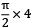× (8.125p + 15.708)

200P = 100P + 125.664 + 6.283(8.125p + 15.708)

100P = 125.664 + 51.05P + 98.69

48.95 P = 224.354

P = 4.58 N/mm2

QUESTION: 5

Circumferential stress in a cylindrical steel boiler shell under internal pressure is 80MPa. Young’s modulus of elasticity and Poisson’s ratio are respectively 2 × 105 MPa and 0.28. The magnitude of circumferential strain in the boiler shell be

Solution: Circumferential stress or Hoop stress,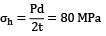Longitudinal stress,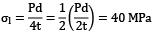Circumferential strain,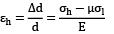=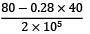= 3.44 × 10−4

QUESTION: 6

When a thin cylinder of diameter ‘d’ and thickness ‘t’ is pressurized with an internal pressure of ‘p’ (1/m is the Poisson’s ratio and E is the modulus of elasticity), then out of the following, which statement is correct?

Solution: For a thin cylinder, Longitudinal stress,

σl =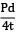Hoop stress, σn =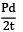Longitudinal strain,

εl =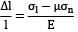=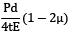or εl =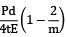where μ =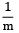Hoop strain,

εh =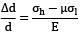=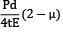or εh =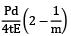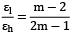QUESTION: 7

A thin walled pressure vessel made of aluminium alloy, 250 mm diameter and 4 mm thick is subjected to an internal pressure of 2.5 N/mm2. Strain gauges mounted on the surface of vessel in circumferential and axial directions give readings of 932 μs and 190 μs respectively, under full pressure, The young’s modulus of the aluminium alloy is _________Gpa

Solution:

P = 2.5 N/mm2

d = 250 mm t = 4 mm εc ,

Circumferential stress

=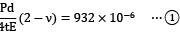εa , axial strain =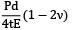= 190 × 10−6 ⋯ ②

Dividing equation ① by equation ②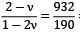= 4.905

or 2 − ν = 4.905 − 9.81 ν 8.81 ν = 2.905

Poisson’s ratio, ν =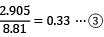From equation ①

E =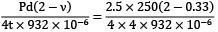= 69994 N/mm2 = 69.99 GPa

Question_Type: 5

QUESTION: 8

A thin cylindrical shell of diameter 200 mm, thickness 8 mm is held between two rigid supports as shown in figure. An internal pressure of 5 N/mm2 is applied on the inner surface of the cylinder. The resultant axial stresses developed in the cylinder (μ = 0.35) is _________MPa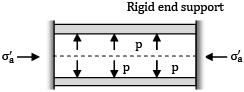(A) 21.0

(B) 22.5

Solution: Stresses developed

σc =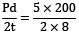= 62.5 N/mm2

σa =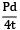= 31.25 N/mm2

Due to rigid end supports, axial strain, εa = 0 Say σa′ is axial compressive stress provided by rigid end supports.

Resultant axial stress, σaR = 31.25 − σa

Axial strain, εa =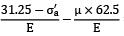= 0

Or 31.25 − σa′ − 0.35(62.5) = 0

σa′ = 31.25 − 21.875

= 9.375 N/mm2

Resultant axial stress, σaR = 31.25 − 9.375 = 21.875 N/mm2

Question_Type: 5

QUESTION: 9

A closed pressure vessel of diameter 120 mm, wall thickness 4 mm is subjected to an internal pressure of 6 N/mm2. The normal stress on an element of cylindrical wall at 30° to the longitudinal axis of cylinder is __________MPa

(A) 78.0

(B) 79.6

Solution: Element ab is inclined at an angle of 30° to the axis 00 of the cylinder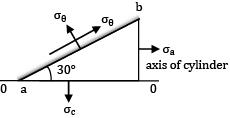Hoop stress,

σc =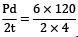= 90 N/mm2

Axial stress, σa =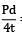=45 N/mm2

Stress on element Normal stress,

σθ =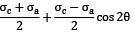==67.5 + 11.25 = 78. 75 N/mm2

Question_Type: 5

QUESTION: 10

A thin cylinder 150 mm internal diameter and 2.5 mm thick has its ends closed by rigid plates and is then filled with water under pressure. When an axial pull of 37 kN is applied to the ends, water pressure is observed to fall by 0.1 N/mm2. The Poisson’s ratio will be (Assume E = 140000 N/mm2, K for water = 2200 N/mm2)

Solution: δp = 0.1 N/mm2

Reduction in volumetric strain of cylinder

=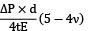Reduction in volumetric strain of water

=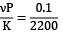Axial force = 37000 N

Axial stress =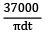=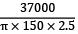= 31.406 N/mm2

Increase in volumetric strain due to pull

=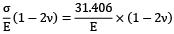Or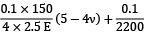=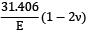Or 1.5(5 − 4ν) +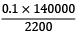= 31.406 (1 − 2ν)

7.5 − 6ν + 6.3636 = 31.406 − 62.812ν

Or 56.812 ν = 31.406 − 6.3636 − 7.5

= 17.5424

Poisson’s ratio,

ν =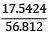= 0.308